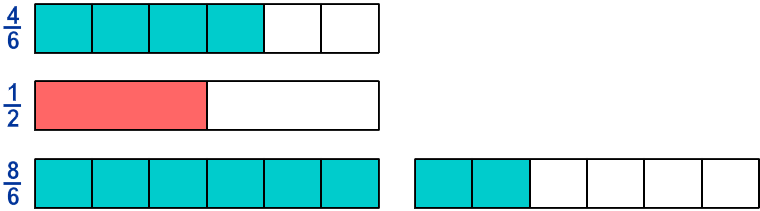# Benchmark Fractions

Rate 0 stars Common Core
Quiz size:
Message preview:
Someone you know has shared Benchmark Fractions quiz with you:

To play this quiz, click on the link below:

https://www.turtlediary.com/quiz/benchmark-fractions.html

Hope you have a good experience with this site and recommend to your friends too.

Login to rate activities and track progress.
Login to rate activities and track progress.

Consider the benchmarks
 1 2
and 1.

Out of these two numbers, let's find which benchmark is between
 4 6
and
 8 6
.

First check the benchmark
 1 2
.

We can represent the fractions
 4 6
,
 1 2
, and
 8 6
as follows:Now let’s compare the shaded areas.

Here, we can notice that
 1 2
is less than
 4 6
and
 8 6
.

So,
 1 2
is not between
 4 6
and
 8 6
.

Now, check the benchmark 1.

We can represent the fractions
 4 6
, 1 and
 8 6
as follows:Now let’s compare the shaded areas.

Here, we can notice that 1 is greater than
 4 6
and less than
 8 6
.
So, 1 is between
 4 6
and
 8 6
.

ds

A B C D E F G H I J K L M N O P Q R S T U V W X Y Z